# Sensors - Why Op Amps? - EdsCave

Go to content

## Sensors - Why Op Amps?

Sensors

The Humble Op-Amp

Operational amplifiers, commonly called op-amps, have become ubiquitous in modern analog design. You can buy op-amps for use in your discrete PCB designs, and chip designers also use them as internal building blocks for analog IC's.  These devices were not always part of the analog designer's toolkit.  Beyond issues of availability and cost, moving from the discrete transistor/tube design philosophy to one based on the opamp required a major paradigm shift.

It's Easy to Amplify

It's not all that difficult to build amplifiers out of discrete transistors. Once upon a time, almost all amplifiers were made from discrete transistors, and further back, they were made from vacuum tubes. Because transistors (and tubes) were once very expensive compared to resistors and capacitors, a big part of 'good design practice' revolved around minimizing the number of transistors or tubes in a given design. This resulted in some very simple amplifier circuits, such as the one shown below.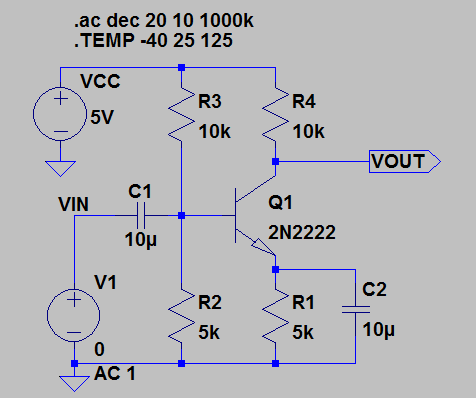A Single-Transistor Amplifier

This single-transistor amplifier provides a gain of 38dB (Vout/Vin ~ 80).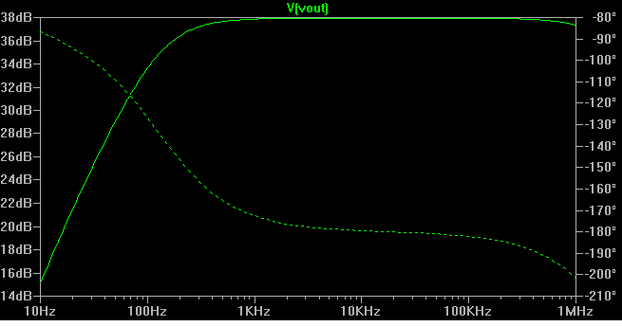Frequency/Phase Response of Above Amplifier

If more gain is desired, there are two basic approaches to getting it. First, one can tweak component values and/or transistor models in the above circuit (2N2222 is the canonical 'generic' discrete transistor) to get minor increases in gain, possibly of values up to a few hundred.  The other way is to cascade multiple copies of the circuit. The latter approach will in theory let you achieve arbitrarily high gains. In reality, however, effects like signal feedback through the power supply, and intrinsic device noise (both from the transistors and passive components) will place some practical limits on just how high you can crank up the gain 'knob' on your circuit design before it either misbehaves (e.g. oscillates) or the amplified internal noise swamps out any signal.

Although it is easy to get gain with simple circuits like the one above, there are several prices to pay. The first is that the circuit may not be all that stable with respect to environmental factors like temperature or operating supply voltage, or part-to-part variation. In simulation (all of the plots shown are from the LTSPICE circuit simulator) , we can specify resistors to be exactly the value we want, the power supply to be exactly 5.0000... volts, and the operating temperature to be exactly +25C.  When we try to implement the circuit in the real world, however, we will find that all of these variables can and do vary, and ways we would often not like, and the circuit performance varies with them.  For example, consider the effect of just changing the temperature over the range of -40C to +125C.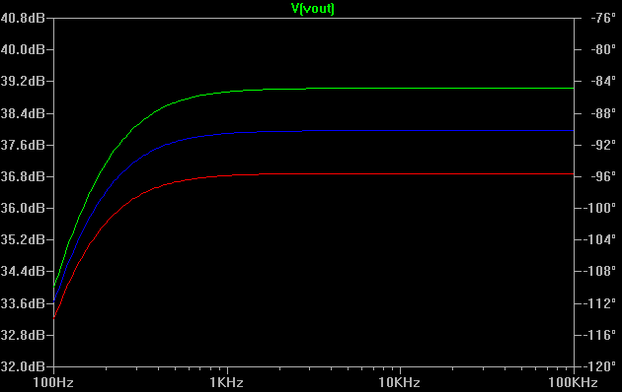Gain vs. Temperature Detail of Simple Amplifier - About 3dB variation from -40C to +125C

The gain changes about 3dB between -40C and +125C - which is about a factor of 30%.  And this is from the LTSPICE simulator, which for this analysis is set up to  assume that temperature only affects the transistor - all of the other components (resistors, capacitors, power supply) are still being modeled as ideal devices that are completely oblivious to temperature effects.

Another issue with this circuit is that the gain also varies as a function of the load which it is driving. The simulations above assume zero load, which might be reasonably approximated by a scope probe (10MegOhms).  If one attempts to drive a load of 10 kohms, which is not an unreasonably low value for many applications, one will discover that the gain drops from 28dB to 32 dB (a factor of 2). If one loads the circuit down with a 1 kohm load, the gain drops from 38dB all the way down to 18 dB - a factor of 10 !

Gain to Burn

The fundamental problem with our simple amplifier is that the gain depends on virtually every aspect of the circuit and how it interacts with its environment.  While there are certainly applications for which this sensitivity might not be a big problem (toys?), there will be others for which it will be a showstopper.  For these more critical applications, there are design techniques that can be used to make the circuit more stable in the face of environmental and component variation. These techniques, however, tend to require more complex design, and more components, which translates into more cost.   Also important is the amount of resources that must go into the design process - clever design and thorough testing is not cheap and certainly not free (despite what the business types may believe).

While incremental performance improvement is often obtainable by incremental design changes, significant improvements typically require a major paradigm shift.  In the case of making stable amplifiers, that paradigm shift comes from employing negative feedback.

In 1927, Harold Black, then working for Bell, came up with the idea of using  feedback as a way to make the amplifier's response more ideal, both in terms of accurately controlling the gain and reducing the distortion.   The basic feedback concept can be seen in the following figure, where the output (Vout) of a primary amplifier A1 is sampled and fed back through a feedback gain A2 to a point where it is added to the incoming signal (Vin).  The sum of these two signals (e) is then used as the input to the primary amplifier.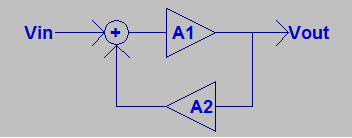Block diagram of Feedback Loop with Forward (A1) and Feedback (A2) Gains.

The math for determining the overall gain of this system requires is straightforward.  The first step is writing an expression for Vout.

Vout = A1 * ( Vin + A2 * Vout)

With a little algebra, this circular expression can be solved for Vout...

Vout = Vin * A1 / (1 - A1 * A2)

In the above expression, the term 'A1 * A2'  has special significance and is what is known as the loop gain.  For most feedback amplifier applications, loop gain has two characteristics:

1) Loop gain is negative (A1 * A2 <  0).  Negative loop gain is required to create negative feedback.  Negative loop gain may be achieved by making either the primary gain negative OR by making the feedback gain negative. If you make both gains negative (or both gains positive), you will end up with a positive feedback loop, which has  qualitatively and often dramatically different behavior than that of a negative feedback loop.

2) For typical applications, loop gain will have an absolute value much greater than 1 (  |A1*A2| >>1 ).  Also the magnitude of A1 will typically be much larger than that of A2, which is usually less than or equal to 1 ( |A2| <=1 ).   In typical implementations, A1 will be realized the circuits containing 'active elements' like transistors or opamps (or vacuum tubes) that can provide significant voltage gain, while A2 will be realized with passive elements like resistors and capacitors that can only attenuate signals.

If you assume that the magnitude of A1 is much greater than A2 (|A1| >> |A2|) and that the loop gain is much greater than one ( |A1*A2| >> 1) , you can approximate the above equation by:

Vout = Vin * 1 / (-A2)

This means that the relationship between Vout and Vin is no longer dependent on the gain of primary amplifier, and is controlled only by the gain of the feedback loop.  Since the feedback loop's gain (or attenuation, depending on how you want to think about it) is usually less than one, it can be implemented with resistors and other passive components which can have good unit-to-unit repeatability and stability.  Controlling gain through the external feedback also makes the design more simple as the system becomes more tolerant of amplifier performance variation.

Let's consider the application of negative feedback to a high-gain amplifier.  In this example, the amplifier has three separate single-transistor inverting gain stages and a emitter follower (Gain ~ 1) used as an output buffer.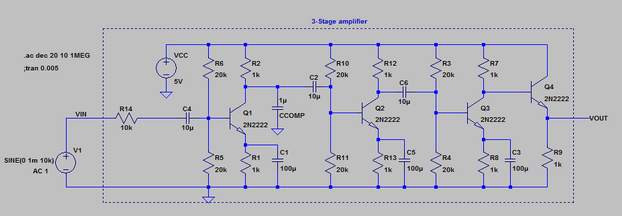Four-stage amplifier with Lots of Gain

The Bode plot for the above amplifier is shown below. One can see that while the gain peaks around 84dB, it varies considerably  over the simulated frequency range.  The degree of gain variation in this amplifier could make it very difficult to use as-is in an actual applications.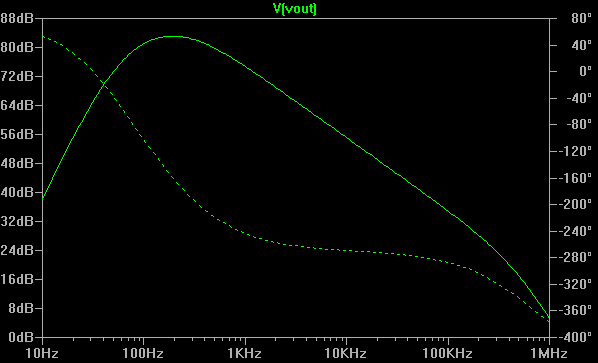Frequency response of Four-stage Amplifier - Varies quite a bit (~75dB) over the Simulated Frequency Range

We are going to make one seemingly very small change to the circuit - adding a feedback signal path from the output back to the input through a single 100k ohm resistor.  By making the connection to the point labeled 'Error Signal',  the feedback path provides a feedback gain of 0.1 (10k/100k).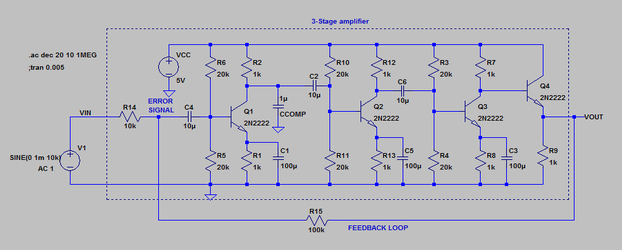Four-stage Amplifier with Addition of Feedback through R15 (A2 = 0.1)

Now if we simulate the modified circuit's performance, we see that the overall system gain (amplifier with feedback) is very close to 20dB, or a factor of 10 over  4 decades of frequency (10Hz-100kHz).  The above change has made the performance dramatically more consistent and predictable over a wide range of frequencies.Response of Amplifier with Feedback - Much more Uniform over Frequency

This is the elegance of using negative feedback in complex systems - it allows us to produce system characteristics that are defined primarily by characteristics of a simple feedback loop. While there are other issues to making feedback work in practice, like maintaining stability (e.g. freedom from spurious oscillation), the main requirement is that we provide excess gain, which can be used to compensate for inconsistencies in the system.

DC-Accurate Amplification

The examples above use amplifiers of the AC-coupled design paradigm, where capacitors are used to decouple amplifier stages. This design style was popular for discrete designs partly because capacitors were cheap (compared to transistors or tubes) and AC coupling stages went a long way to make circuit bias issues go away. The big drawback to AC coupling is that DC signals are not passed through the amplifier, much less amplified. While this might not be a problem in an audio amplifier in a radio, it is a major issue in measurement instrumentation, where the signals to be amplified may be fundamentally DC in nature.

Fortunately, in those situations where DC gain and accuracy is important, there is a circuit building block called the differential pair, one implementation of which is  shown below.  The key to this circuit's operation is splitting a fixed amount of current (I1 current source) between two symmetric amplifier sections.  when the base voltages of Q1 and Q2 are equal, the current (2mA) is split evenly between the two transistors, so each transistor emitter carries 1mA.  Because Q2's base is at 0V (grounded), this balanced condition occurs when Q1's base voltage is also 0V. The two emitter currents (minus a small amount of base current) flow down through their respective collectors, and the load resistors, which provide output voltages V)+ and VO-. In the balanced condition, VO+ and VO- are both 1V for this example.Differential Pair is a Fundamental Building Block for DC Accurate Amplifiers

One feature of the differential pair is that if both input voltages (Q1, Q2 bases) are moved up or down over a limited range (common-mode input range), the balance is maintained. Only when one voltage is higher than the other is the balance disturbed.  For example, if one injects a sine wave into Q1 while leaving Q2 grounded, the following waveforms will be seen on VO+ and VO-.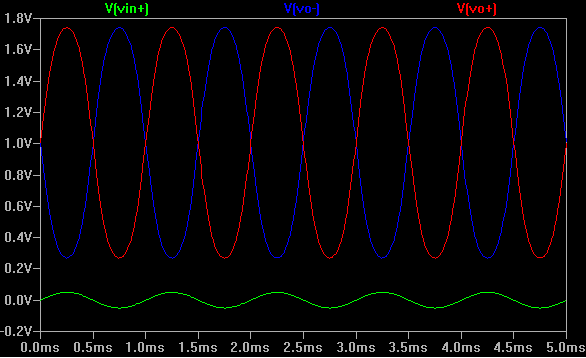Outputs of Differential Pair in response to input signal.

Note that in addition to providing gain, the two outputs are 180 degrees out of phase with each other. The differential pair can therefore be used to provide both positive and negative gain depending on which input the original signal is fed into and which output is tapped.

If we combine a differential pair input stage with a high-gain stage, we get a system building block with the functionality shown in the figure below.  We get a high forward gain useful in making feedback loops, and have both inverting and non-inverting inputs, providing flexibility in how we configure the feedback.  The differential inputs also allow us to reference the amplification function to an arbitrary voltage, such as 0V, by tying the unused input the that voltage.System Description of Opamp

The Figure below shows a highly simplified and idealized opamp circuit. Q1 and Q2 form the differential pair. Q3,Q4, Q5  are a current-subtracting and amplification circuit that take the balanced outputs from Q1 and Q2's collectors and convert them into a single-ended voltage, and also add significant amounts of gain in the process. Finally, Q6 is a buffer (emitter follower circuit) that improves the opamp's ability to drive loads.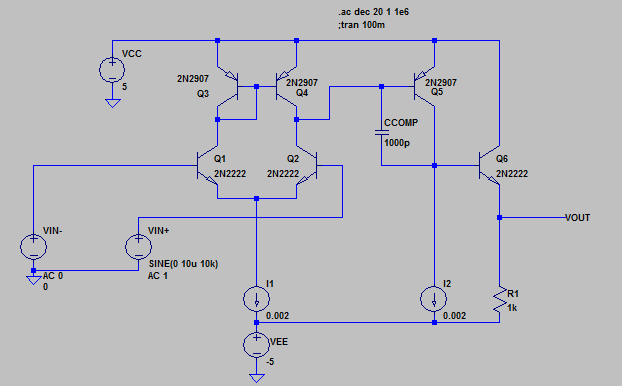Very Idealized Opamp Circuit

As you can see in the Bode plot below, the gain of our simplified opamp is quite high (>110dB), but goes down sharply as frequency increases.  A big part of the variation is due to the presence of CCOMP (1000pF) in the above circuit. This compensation capacitor was added to deliberately reduce gain with increasing frequency - doing so makes it more likely that the feedback loop into which the opamp is embedded will be stable (not oscillate). Many, if not most, commercially available opamps are designed in this way to make them easier to use.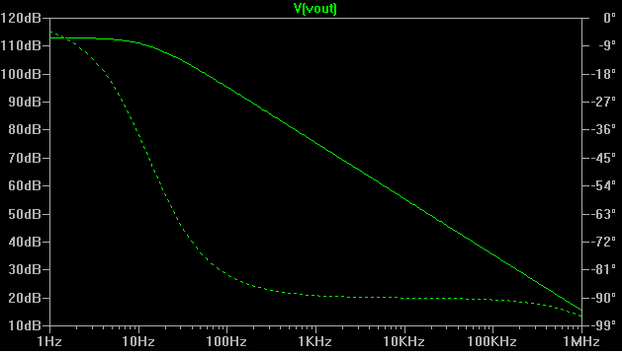Bode Plot of simplified Opamp

Now let's wrap a feedback loop around our simple opamp.  In the circuit below, the input signal is applied to the VIN+ (non-inverting) opamp input, and the feedback is brought into the VIN- terminal,  so the 'A1' forward gain is positive  while the 'A2' feedback gain is negative, even though the feedback loop only contains a 10:1 resistive divider which does not invert the feedback signal in any way by itself.Simplified Opamp with 0.1 Feedback Gain from 9k/1k Resistive Divider

When we simulate the above circuit, we see the Bode plot below - with a nice flat gain of 20dB (10) across near all of the simulated frequency range.  Although not shown (and not available in AC analysis mode), the gain will also remain flat down to 0Hz, or DC.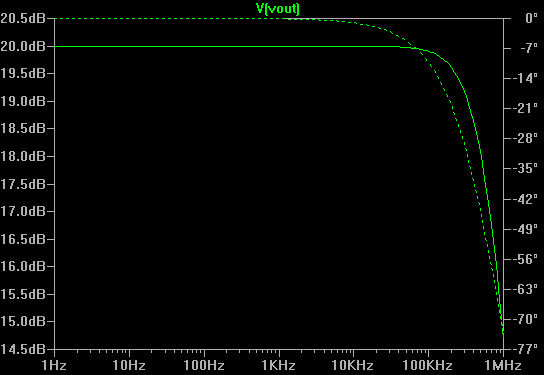Bode Plot of Simple Opamp with Feedback Loop Wrapped Around it

Running a time-domain simulation (plots below) yields a bit more insight into how the circuit behaves.  The VIN+ and VIN- signals are virtually identical, while the VOUT signal is similar, but ten times the magnitude.  The VIN+ signal is set by the external signal source.  What is happening is that because the opmap is set up in a negative feedback system, its output voltage will swing to whatever value it can to try to keep the VIN- signal equal to the VIN+ signal - or a zero differential voltage. It is important to note that the opamp can't directly control the VIN- input, but can only influence it through the feedback.  Because VIN- is derived from a 10:1 voltage divider connected to the VOUT terminal, the VOUT terminal needs to swing a factor of 10 larger voltage than that required at VIN- to match VIN+.  Also note that the output voltage swings symmetrically around 0V, as does VIN+.   For the case of a DC input, the opamp will attempt to produce an VOUT voltage 10X that of the VIN+ input voltage.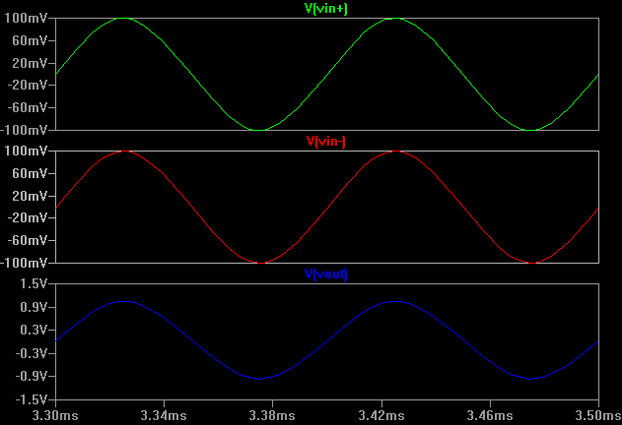Time-domain behavior of Opamp with Feedback

Feedback Cures a Host of Ills

We have shown how negative feedback can tame an amplifier's wildly varying frequency response. It can also help cure linearity problems as well.  Consider the simple power amplifier circuit shown below.  Such a circuit might be used as the final stage in a headphone driver.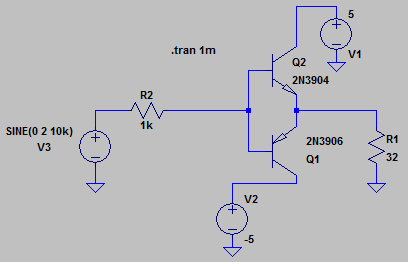Push-Pull Power Amplifier Stage

The big problem with above circuit, however, is that it is highly non-linear, especially when the signals are not very large.  The time-domain simulation below contrasts the signal going in (green) and what comes out (red).  The technical term for this particular type of non-linearity is crossover distortion , and results because there is a point on each waveform when neither transistor is driven hard enough to become active.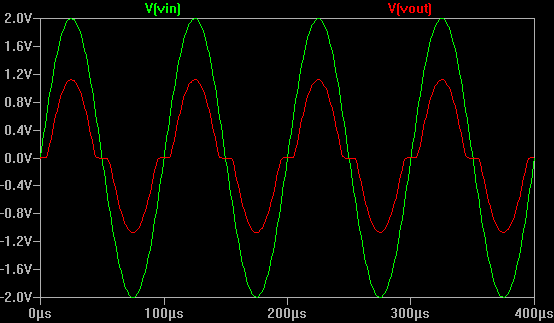Push-Pull Amplifier Distortion

If you played a typical music track through this output stage, it would probably sound pretty horrible, unless your idea of good music involves lots of distortion effects.  One way of reducing this problem is to put the amplifier in a feedback loop with an opamp, as shown below. This time to simplify things, we are going to just use one of the LTSPICE library opamps, an LT1013, as opposed to building one ourselves.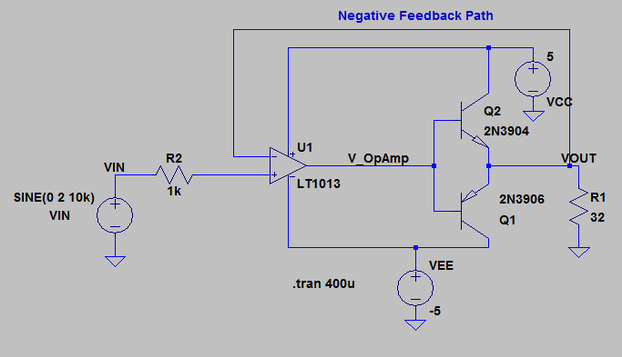Opamp and feedback used to Reduce Crossover Distortion.

In the time-domain upper plot panel shown below, you can see that the VOUT signal now substantially tracks the VIN signal, and that the distortion has been greatly reduced.  Based on the previous example, this is not that surprising, considering that VIN is connected to the opamp's VIN+ terminal and VOUT is connected to the VIN- terminal.  What is a little more surprising is what the opamp's output is doing. If you look at the lower plot panel you can see the opamp's output superimposed on VOUT. In this case the feedback information is being used by the opamp to pre-distort the drive to the power amplifier in just the right way to generate the desired (minimally distorted) signal at the power amplifier's output.  When used properly, negative feedback can produce almost magical-seeming results!Behavior at Key Points in Power amplifier with Feedback Loop

System Design vs. Circuit Design

When doing design at the transistor level, there are two common concerns - the behavior of individual components, and the interactions of those components. Individual component models, even in highly idealized form, can be quite complex.  Because of loading effects, inter-component interactions are also quite complex - from a mathematical standpoint what you have to deal with are sets of simultaneous non-linear equations.  And this is just to analyze 'DC Bias Points'.  Even though an analog circuit like an opamp may only have 30-50 transistors,  doing transistor-level analog design at this level of complexity can be quite challenging.

By being able to 'wrap-up' the functionality of an opamp into a re-usable module, we can hide a great deal of design complexity.  By using opamps and feedback networks in our designs, to some degree we also sweep a lot of complexity under the rug. This is partly because the opamp concept allows us to hide a lot of the complexity of active devices - by using opamps, we can define system properties primarily using better behaved and comparatively simple passive devices like resistors and capacitors. Another simplification is trading bi-direction interactions that result from loading for unidirectional signal-flow type interactions - by designing subsystems so that they have very high input impedance and very low output impedance modularizes them and makes it easier to connect them together into more complex systems with predictable behavior.  In short, the opamp/feedback paradigm gives analog designers a tool for designing complex circuits at the system level, and letting them focus on detailed circuit behavior on the more critical points in their creations.

29 NOV 2016